## The Golden Ratio

by | Apr 2, 2018Golden Ratio Spiral

Today’s IFOD is about “The Golden Ratio” otherwise known as “phi.” The golden ratio is pretty interesting, but first, let’s discuss reproduction of bunnies (or you can just skip down to the meat of the golden ratio below):

In 1202 Leonardo de Pisa, popularly known as Fibonacci, posed the following question:  “A certain man put a pair of rabbits in a place surrounded on all sides by a wall.  How many pairs of rabbits can be produced from that pair in a year if it is supposed that every month each pair begets a new pair which from the second month on becomes productive?”  If you assume that each pair consists of one male and one female and that they are able to procreate after their first month and there is a one-month gestation period and that the bunnies are monogamous, here’s what you get:

• You begin with a pair of baby rabbits at the beginning of the first month
• A month later that pair of bunnies is now adult and the following month they have their first pair of bunnies.
• So, after the first month you have one pair.  After two months you have one pair.  After three months there are two pair.  In the fourth month the original pair gives birth again, while the new pair from month three grows to adulthood.  So, there are three pair.  Following still?  In the fifth month the original couple produces yet another pair, and now the first set of offspring produce offspring.  Now there are five pair.  The next month there are eight pair. And so on.Bunnies in love. Also part of a Fibonnaci Sequence!

This sequence of numbers is referred to as the Fibonacci Sequence.  Not because this is necessarily how rabbits reproduce, but because of the importance of the sequence that the rabbit example produces:

1, 1, 2, 3, 5, 8, 13, 21, 34, 55, 89, 144, 233 . . . .

Ignoring the rabbit aspect of the example above, you create the Fibonacci sequence by adding together the first two numbers to get the third, and then the second and third to get the fourth, and so on.

Now for the Golden Ratio: If you take the ratio of consecutive Fibonacci numbers, the resulting ratio settles down into what is known as “the Golden Ratio”, or 1.6180339887498948482… Example:

• 1/1 = 1
• 2/1 = 2
• 3/2 = 1.5
• 5/3 = 1.6666…
• 8/5 = 1.6
• 13/8 = 1.625
• 21/13 = 1.61538…
• 34/21 = 1.61904…
• 55/34 = 1.61764…
• 89/55 = 1.61818…
• And so on . . .

We find the golden ratio when a line is segmented so that the whole length of the line divided by the long part is also equal to the long part divided by the short part.From “mathisfun.com”

Golden Rectangle is one where the ratio between the length and height is Phi (so, if a = 1, a+b = 1.618. . . ).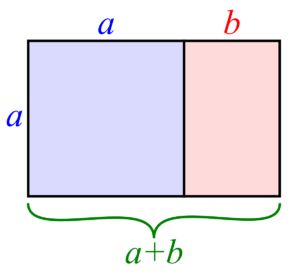In this figure, the pink rectangle is a “golden rectangle” while the blue square is just a square. Also, the combination of the square and the pink rectangle creates a “golden rectangle” as well.

The Golden Ratio appears all throughout nature, in art, architecture, music and more.  Often rectangles in Golden Ratio proportions seem most pleasing to us, as do triangles with Phi proportions. Buildings constructed using the Golden Ratio tend to be more aesthetically pleasing. The Parthenon makes great use of the Golden Ratio, as does the Great Pyramid at Giza, many Gothic cathedrals,  as well as many modern buildings. The Christian Cross is often represented so that the horizontal part of the cross intersects the vertical at the the Golden Ratio proportion.

Why do we like things with the Golden Ratio? From The Atlantic: “According to Adrian Bejan, professor of mechanical engineering at Duke University, the human eye is capable of interpreting an image featuring the golden ratio faster than any other….Whether intentional or not, the ratio represents the best proportions to transfer to the brain. This is the best flowing configuration for images from plane to brain and it manifests itself frequently in human-made shapes that give the impression they were ‘designed’ according to the golden ratio,” said Bejan.

Here are some examples of the Golden Ratio from nature:

“The number of petals in a flower consistently follows the Fibonacci sequence. Famous examples include the lily, which has three petals, buttercups, which have five (pictured below), the chicory’s 21, the daisy’s 34, and so on (all Fibonacci numbers).” (from Gizmodo).Seed heads in daisies and sunflowers follow the Fibonacci sequence (e.g., 21 rows in one direction, 34 in the other direction):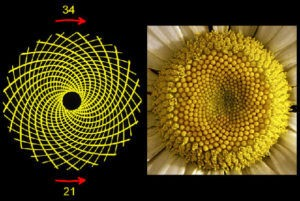Source: Gizmodo

Branching in trees typically follow Fibonacci sequence: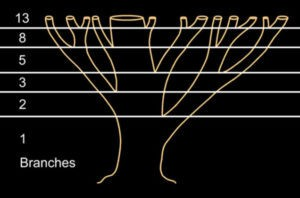Animal bodies: “The measurement of the human navel to the floor and the top of the head to the navel is the Golden ratio. But we are not the only examples of the Golden ratio in the animal kingdom; dolphins, starfish, sand dollars, sea urchins, ants and honeybees also exhibit the proportion.” (from Live Science). The size of the hand vs. forearm vs. whole arm also fits the ratio.A DNA molecule measures 34 angstroms by 21 angstroms at each full cycle of the double helix spiral. In the Fibonacci series, 34 and 21 are successive numbers.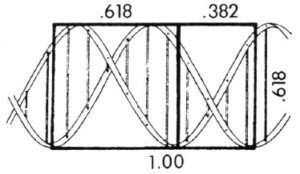The arrangement of a pine cone: “The spiral pattern of the seed pods spiral upward in opposite directions. The number of steps the spirals take tend to match Fibonacci numbers.” (From Live Science)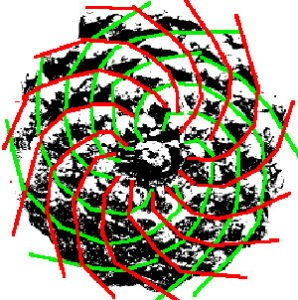The shape of spiral galaxies:Shells often reflect Fibonacci sequences: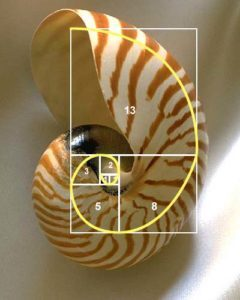The shape of Hurricanes: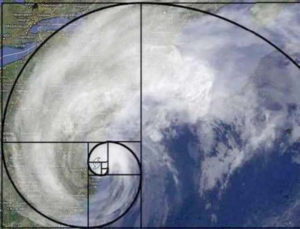Among other things. Have a nice Monday!

This site uses Akismet to reduce spam. Learn how your comment data is processed.## Archives## Subscribe To The IFOD

Get the Interesting Fact of the Day delivered twice a week. Plus, sign up today and get Chapter 2 of John's book The Uncertainty Solution to not only Think Better, but Live Better. Don't miss a single post!